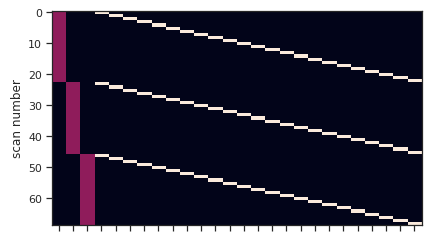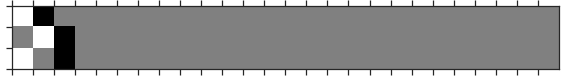# One-way repeated anova in nistats

Hi gang,

I hope y’all are doing ok in these difficult times.

@jeromedockes and I had some “fun” endeavors running
a one-way repeated anova in nistats (still using the archived version, as the new release within nilearn is not here yet).

The design: I have 3 conditions and 23 participants. Based on that I want to investigate the effect of condition, that is the difference between the three levels of the factor condition, using a one-way repeated anova.
Here’s the design matrix:I set my contrast up as follows:

`eoi = np.vstack(([1, 0, -1] +  * n_subjects, [0, 1, -1] +  * n_subjects, [1, 0, -1] +  * n_subjects))`

which results in:

`plot_contrast_matrix(eoi, second_level_design)`Looks ok, but running the model results in the following error:

``````second_level_model.compute_contrast(
eoi,
second_level_stat_type='F',
output_type='z_score')
``````

`LinAlgError: Singular matrix` (here)

Does anyone have any idea what might go wrong (anyone as in @bthirion maybe)?

Cheers, Peer

Based on this nistats example we saw I believe the second-level contrast should be

``````1 0 0 0 ... 0
0 1 0 0 ... 0
0 0 1 0 ... 0
``````

(3 rows, 3 + n_subjects columns). but the docstrings of `FirstLevelModel` and `SecondLevelModel` give no information about 2-dimensional contrast vectors (for “F” stat type, as used in the example)

Yes, the matrix singular because the participants columns sum to 1, as do the conditions columns.
2 possibilities:

• remove the column of one participant
• remove the column of one condition (assuming that this is a baseline). Implicitly the other columns will represent a condition vs this baseline.
Does that sound OK ?

or remove the third row of contrast matrix which is redundant (and thus makes the matrix singular)

Hi @bthirion and @jeromedockes,

thx for the pointers. I decided to go with @jeromedockes suggestion, as we discussed that the `effect of interest` described here refers to testing `condition 1 = condition 2 = condition 3 = 0` and the using only the first two rows to `condition 1 = condition 2 = condition 3`.

Thx again, cheers, Peer